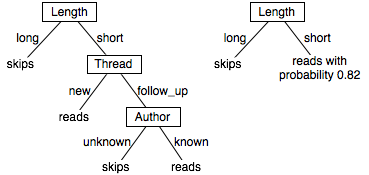### 7.3.1 Learning Decision Trees

A decision tree is a simple representation for classifying examples. Decision tree learning is one of the most successful techniques for supervised classification learning. For this section, assume that all of the features have finite discrete domains, and there is a single target feature called the classification. Each element of the domain of the classification is called a class.

A decision tree or a classification tree is a tree in which each internal (non-leaf) node is labeled with an input feature. The arcs coming from a node labeled with a feature are labeled with each of the possible values of the feature. Each leaf of the tree is labeled with a class or a probability distribution over the classes.

To classify an example, filter it down the tree, as follows. For each feature encountered in the tree, the arc corresponding to the value of the example for that feature is followed. When a leaf is reached, the classification corresponding to that leaf is returned.Figure 7.4: Two decision trees

Example 7.5: Figure 7.4 shows two possible decision trees for the example of Figure 7.1. Each decision tree can be used to classify examples according to the user's action. To classify a new example using the tree on the left, first determine the length. If it is long, predict skips. Otherwise, check the thread. If the thread is new, predict reads. Otherwise, check the author and predict read only if the author is known. This decision tree can correctly classify all examples in Figure 7.1.

The tree on the right makes probabilistic predictions when the length is short. In this case, it predicts reads with probability 0.82 and so skips with probability 0.18.

A deterministic decision tree, in which all of the leaves are classes, can be mapped into a set of rules, with each leaf of the tree corresponding to a rule. The example has the classification at the leaf if all of the conditions on the path from the root to the leaf are true.

Example 7.6: The leftmost decision tree of Figure 7.4 can be represented as the following rules:
skips ←long.